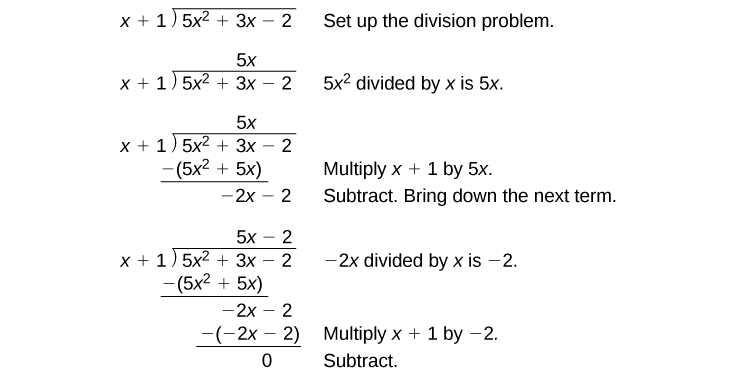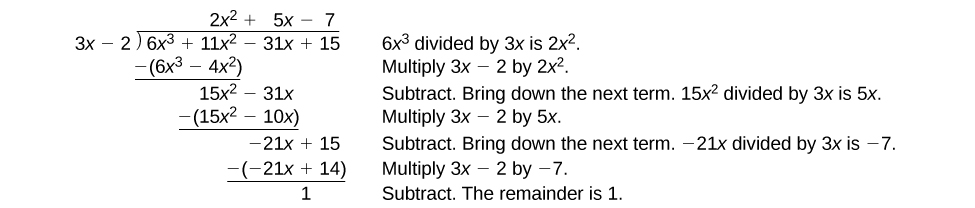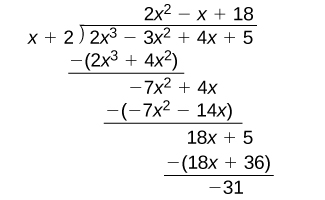# 5.4 Dividing polynomials  (Page 2/6)

 Page 2 / 6

Given a polynomial and a binomial, use long division to divide the polynomial by the binomial.

1. Set up the division problem.
2. Determine the first term of the quotient by dividing the leading term of the dividend by the leading term of the divisor.
3. Multiply the answer by the divisor and write it below the like terms of the dividend.
4. Subtract the bottom binomial    from the top binomial.
5. Bring down the next term of the dividend.
6. Repeat steps 2–5 until reaching the last term of the dividend.
7. If the remainder is non-zero, express as a fraction using the divisor as the denominator.

## Using long division to divide a second-degree polynomial

Divide $\text{\hspace{0.17em}}5{x}^{2}+3x-2\text{\hspace{0.17em}}$ by $\text{\hspace{0.17em}}x+1.$The quotient is $\text{\hspace{0.17em}}5x-2.\text{\hspace{0.17em}}$ The remainder is 0. We write the result as

$\frac{5{x}^{2}+3x-2}{x+1}=5x-2$

or

$5{x}^{2}+3x-2=\left(x+1\right)\left(5x-2\right)$

## Using long division to divide a third-degree polynomial

Divide $\text{\hspace{0.17em}}6{x}^{3}+11{x}^{2}-31x+15\text{\hspace{0.17em}}$ by $\text{\hspace{0.17em}}3x-2.\text{\hspace{0.17em}}$There is a remainder of 1. We can express the result as:

$\frac{6{x}^{3}+11{x}^{2}-31x+15}{3x-2}=2{x}^{2}+5x-7+\frac{1}{3x-2}$

Divide $\text{\hspace{0.17em}}16{x}^{3}-12{x}^{2}+20x-3\text{\hspace{0.17em}}$ by $\text{\hspace{0.17em}}4x+5.\text{\hspace{0.17em}}$

$4{x}^{2}-8x+15-\frac{78}{4x+5}$

## Using synthetic division to divide polynomials

As we’ve seen, long division of polynomials can involve many steps and be quite cumbersome. Synthetic division is a shorthand method of dividing polynomials for the special case of dividing by a linear factor whose leading coefficient is 1.

To illustrate the process, recall the example at the beginning of the section.

Divide $\text{\hspace{0.17em}}2{x}^{3}-3{x}^{2}+4x+5\text{\hspace{0.17em}}$ by $\text{\hspace{0.17em}}x+2\text{\hspace{0.17em}}$ using the long division algorithm.

The final form of the process looked like this:There is a lot of repetition in the table. If we don’t write the variables but, instead, line up their coefficients in columns under the division sign and also eliminate the partial products, we already have a simpler version of the entire problem.Synthetic division carries this simplification even a few more steps. Collapse the table by moving each of the rows up to fill any vacant spots. Also, instead of dividing by 2, as we would in division of whole numbers, then multiplying and subtracting the middle product, we change the sign of the “divisor” to –2, multiply and add. The process starts by bringing down the leading coefficient.We then multiply it by the “divisor” and add, repeating this process column by column, until there are no entries left. The bottom row represents the coefficients of the quotient; the last entry of the bottom row is the remainder. In this case, the quotient is $\text{\hspace{0.17em}}2{x}^{2}–7x+18\text{\hspace{0.17em}}$ and the remainder is $\text{\hspace{0.17em}}–31.\text{\hspace{0.17em}}$ The process will be made more clear in [link] .

## Synthetic division

Synthetic division is a shortcut that can be used when the divisor is a binomial in the form $\text{\hspace{0.17em}}x-k\text{\hspace{0.17em}}$ where $\text{\hspace{0.17em}}k\text{\hspace{0.17em}}$ is a real number. In synthetic division    , only the coefficients are used in the division process.

Given two polynomials, use synthetic division to divide.

1. Write $\text{\hspace{0.17em}}k\text{\hspace{0.17em}}$ for the divisor.
2. Write the coefficients of the dividend.
3. Bring the lead coefficient down.
4. Multiply the lead coefficient by $\text{\hspace{0.17em}}k.\text{\hspace{0.17em}}$ Write the product in the next column.
5. Add the terms of the second column.
6. Multiply the result by $\text{\hspace{0.17em}}k.\text{\hspace{0.17em}}$ Write the product in the next column.
7. Repeat steps 5 and 6 for the remaining columns.
8. Use the bottom numbers to write the quotient. The number in the last column is the remainder and has degree 0, the next number from the right has degree 1, the next number from the right has degree 2, and so on.

root under 3-root under 2 by 5 y square
The sum of the first n terms of a certain series is 2^n-1, Show that , this series is Geometric and Find the formula of the n^th
cosA\1+sinA=secA-tanA
why two x + seven is equal to nineteen.
The numbers cannot be combined with the x
Othman
2x + 7 =19
humberto
2x +7=19. 2x=19 - 7 2x=12 x=6
Yvonne
because x is 6
SAIDI
what is the best practice that will address the issue on this topic? anyone who can help me. i'm working on my action research.
simplify each radical by removing as many factors as possible (a) √75
how is infinity bidder from undefined?
what is the value of x in 4x-2+3
give the complete question
Shanky
4x=3-2 4x=1 x=1+4 x=5 5x
Olaiya
hi can you give another equation I'd like to solve it
Daniel
what is the value of x in 4x-2+3
Olaiya
if 4x-2+3 = 0 then 4x = 2-3 4x = -1 x = -(1÷4) is the answer.
Jacob
4x-2+3 4x=-3+2 4×=-1 4×/4=-1/4
LUTHO
then x=-1/4
LUTHO
4x-2+3 4x=-3+2 4x=-1 4x÷4=-1÷4 x=-1÷4
LUTHO
A research student is working with a culture of bacteria that doubles in size every twenty minutes. The initial population count was  1350  bacteria. Rounding to five significant digits, write an exponential equation representing this situation. To the nearest whole number, what is the population size after  3  hours?
v=lbh calculate the volume if i.l=5cm, b=2cm ,h=3cm
Need help with math
Peya
can you help me on this topic of Geometry if l help you
litshani
( cosec Q _ cot Q ) whole spuare = 1_cosQ / 1+cosQ
A guy wire for a suspension bridge runs from the ground diagonally to the top of the closest pylon to make a triangle. We can use the Pythagorean Theorem to find the length of guy wire needed. The square of the distance between the wire on the ground and the pylon on the ground is 90,000 feet. The square of the height of the pylon is 160,000 feet. So, the length of the guy wire can be found by evaluating √(90000+160000). What is the length of the guy wire?
the indicated sum of a sequence is known as
how do I attempted a trig number as a starter
cos 18 ____ sin 72 evaluateByByBy Brooke DelaneyBy OpenStaxBy Madison ChristianBy OpenStaxBy Yasser IbrahimBy Stephen VoronBy Madison ChristianBy Saylor FoundationBy OpenStaxBy Stephen Voron